Next: The Simplex Algorithm Up: An algebraic characterization of Previous: Basic Feasible Solutions: An

#### Example:

Returning to the prototype example, it is easy to see that its ``standard form'' formulation is as follows: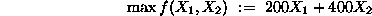s.t.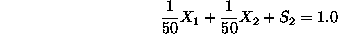This formulation involves four variables and two technological constraints. Therefore, any basic solution will be defined by selecting a basis of two variables, with the remaining two being set equal to zero. For example, selecting as basis the variable set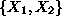implies that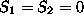(since they are the remaining non-basic variables). This further implies that the considered basic solution corresponds to the extreme point of the LP feasible region defined by the binding of the two technological constraints. Finally, the values of the two basic variables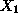and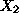are obtained by solving the system of equations: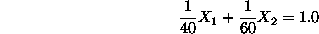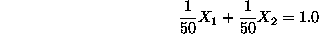which results from the technological constraints in ``standard form'', by eliminating the non-basic variables.

On the other hand, consider the extreme point B of the feasible region, which is the optimal solution of this example LP. It has been already shown (cf. previous example) that this point is defined by the binding of the second technological constraint and the sign restriction imposed on variable. Hence, at this point,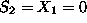, and the corresponding basis consists of variablesand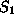. To compute the values for these variables, we solve the system of equations: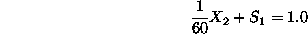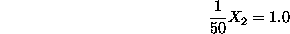Finally, the basic solution defined by the basis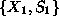implies that, and therefore, the corresponding point on the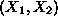-plane, F, is defined by the binding of second technological constraint and the sign restriction of variable. Solving the system of equations: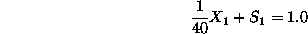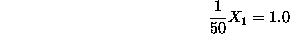we obtain: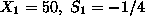. This basic solution is not feasible, which is also reflected in Figure 2 by the fact that point F is not an extreme point of the feasible region.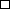.

In the example above, extreme points B and C are adjacent, in the sense that they are linked by one edge of the feasible region. This reflects in the structure of the corresponding bases by the fact that they differ in only one binding constraint. This observation generalizes to the n-dimensional case: extreme points connected by ``edges'' of the feasible region have n-1 common binding constraints, and therefore, their corresponding bases will differ in one variable only. Hence, we have the following definition: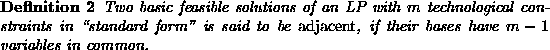The characterization of the extreme points of the feasible region of an LP as basic feasible solutions for its ``standard form'' representation provides the analytical means for organizing the search for an optimal extreme point performed by the Simplex algorithm. The details of this algorithm is the topic of the next section.Next: The Simplex Algorithm Up: An algebraic characterization of Previous: Basic Feasible Solutions: An

UAL Data
Fri Jun 20 15:03:05 CDT 1997